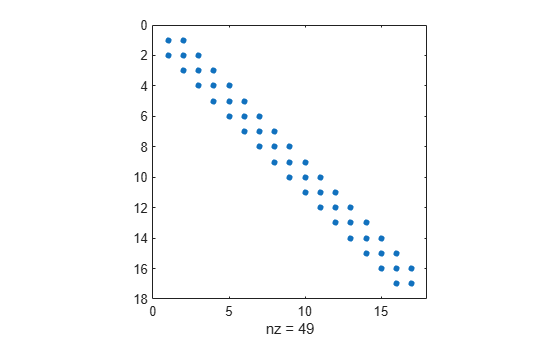# det

Matrix determinant

## Syntax

``d = det(A)``

## Description

example

````d = det(A)` returns the determinant of square matrix `A`.```

## Examples

collapse all

Create a 3-by-3 square matrix, `A`.

`A = [1 -2 4; -5 2 0; 1 0 3]`
```A = 3×3 1 -2 4 -5 2 0 1 0 3 ```

Calculate the determinant of `A`.

`d = det(A)`
```d = -32 ```

Examine why the determinant is not an accurate measure of singularity.

Create a 10-by-10 matrix by multiplying an identity matrix, `eye(10)`, by a small number.

`A = eye(10)*0.0001;`

The matrix `A` has very small entries along the main diagonal. However, `A` is not singular, because it is a multiple of the identity matrix.

Calculate the determinant of `A`.

`d = det(A)`
```d = 1.0000e-40 ```

The determinant is extremely small. A tolerance test of the form `abs(det(A)) < tol` is likely to flag this matrix as singular. Although the determinant of the matrix is close to zero, `A` is actually not ill conditioned. Therefore, `A` is not close to being singular. The determinant of a matrix can be arbitrarily close to zero without conveying information about singularity.

To investigate if `A` is singular, use either the `cond` or `rcond` functions.

Calculate the condition number of `A`.

`c = cond(A)`
```c = 1 ```

The result confirms that `A` is not ill conditioned.

Examine a matrix that is exactly singular, but which has a large nonzero determinant. In theory, the determinant of any singular matrix is zero, but because of the nature of floating-point computation, this ideal is not always achievable.

Create a 13-by-13 diagonally dominant singular matrix `A` and view the pattern of nonzero elements.

```A = diag([24 46 64 78 88 94 96 94 88 78 64 46 24]); S = diag([-13 -24 -33 -40 -45 -48 -49 -48 -45 -40 -33 -24],1); A = A + S + rot90(S,2); spy(A)````A` is singular because the rows are linearly dependent. For instance, `sum(A)` produces a vector of zeros.

Calculate the determinant of `A`.

`d = det(A)`
```d = 1.1127e+05 ```

The determinant of `A` is quite large despite the fact that `A` is singular. In fact, the determinant of `A` should be exactly zero! The inaccuracy of `d` is due to an aggregation of round-off errors in the MATLAB® implementation of the LU decomposition, which `det` uses to calculate the determinant. This result demonstrates a few important aspects of calculating numeric determinants. See the Limitations section for more details.

## Input Arguments

collapse all

Input matrix, specified as a square numeric matrix.

Data Types: `single` | `double`
Complex Number Support: Yes

## Limitations

Avoid using `det` to examine if a matrix is singular because of the following limitations. Use `cond` or `rcond` instead.

LimitationResult

The magnitude of the determinant is typically unrelated to the condition number of a matrix.

The determinant of a matrix can be arbitrarily large or small without changing the condition number.

`det` uses the LU decomposition to calculate the determinant, which is susceptible to floating-point round-off errors.

The determinant calculation is sometimes numerically unstable. For example, `det` can produce a large-magnitude determinant for a singular matrix, even though it should have a magnitude of 0.

## Algorithms

`det` computes the determinant from the triangular factors obtained by Gaussian elimination with the `lu` function.

```[L,U] = lu(X) s = det(L) % This is always +1 or -1 det(X) = s*prod(diag(U))```

## Version History

Introduced before R2006a